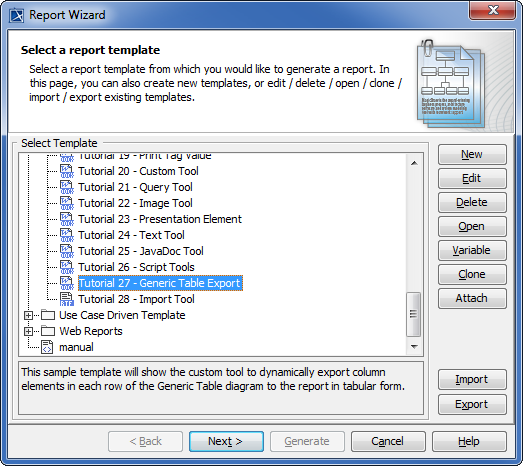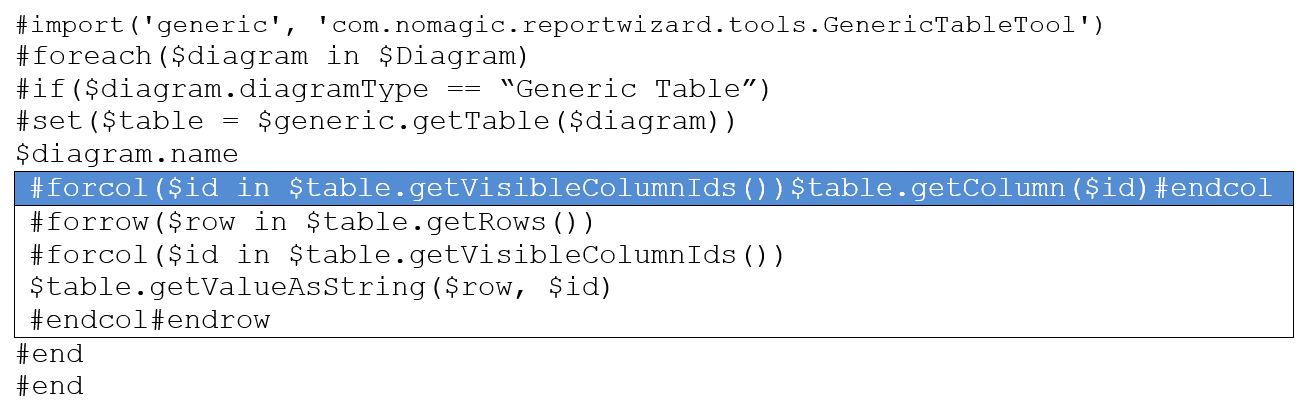##### Page tree

MagicDraw 19.0 LTR SP3 Documentation

The code in this section demonstrates some scenarios with a Generic Table tool. The code may not be executable or may not return the correct result. This is only the idea of how to use the Generic Table tool and how it works.

The code shown below prints the row, column, and its value in the form of an HTML table.

```#foreach(\$diagram in \$project.getDiagrams("Generic Table"))
<table>
<tr>
#set(\$table = \$generic.getTable(\$diagram))
<td>#</td>
#foreach(\$colName in \$table.columnNames)
<td>\$colName</td>
#end
</tr>
#foreach(\$row in \$table.rows)
<tr>
<td>\$velocityCount</td>
#foreach(\$col in \$table.columnIds)
<td>\$table.getValueAsString(\$row, \$col)</td>
#end
</tr>
#end
</table>
#end```

#### How to learn more about the GenericTableTool

1. From MagicDraw main menu choose Tools > Report Wizard.
2. In the Select Template pane, choose Tutorial > Tutorial 27 - Generic Table Export.
3. Click Next, and choose Built-in for Select Report Data.
4. Click Next again, Select Element Scope, and then click Next again. Select output options and click Generate.
######The Generic Table Export dialog.

You can retrieve other diagram table types by using the complete code shown below for getting values from the Generic Table:###### The complete code for getting values from the Generic Table.

Note

You can customize line of code, e.g., by adding diagram table types to get the Metric Table or Instance Table, as shown below:

`#if(\$diagram.diagramType == “Metric Table” || \$diagram.diagramType == “Instance Table”)`
• No labels